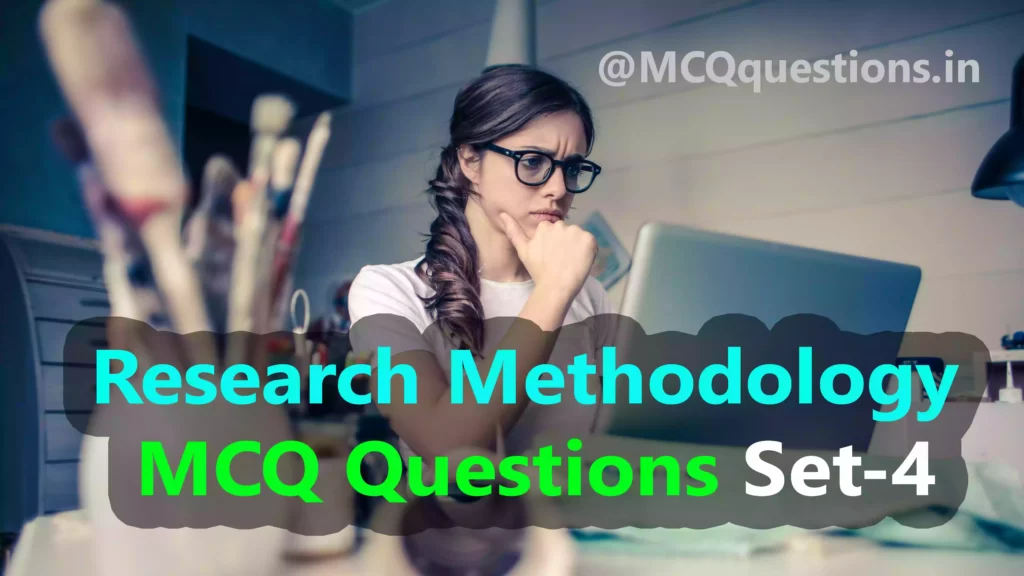# Research Methodology MCQ Questions Set-4

## Research Methodology MCQ

1. The evolution of operation research could be associated within well-known development of

(A) Industrial organization

(B) Institutional organization

(C) Small scale organization

`Answer (A) Industrial organization`

2. The problem and techniques can be classified broadly into

(A) Inventory control

(B) Game theory

(C) Network analysis

(D)All of these

`Answer (D) All of these`

3. Which of the following is/are essential requirement/s to carry out a successful research

Process?

(A) Planning

(B) Guidance

(C) Experts

(D) All of these

`Answer (D) All of these`

4. Which of the following has a great impact mind of the researcher?

(A) References

(B) Finance

(C) Journals

(D) Library

`Answer (A) References`

5. Which of the following is the first step in a research process?

(A) Selecting a topic

(B) Formulating research problem

(C) Development of a hypothesis

(D) None of the above

`Answer (B) Formulating research problem`

6. Hypothesis relate generally or specifically

(A) Variables to variables

(B) Constant to variables

(C) Variables to constant

(D) Constant to constant.

`Answer (A) Variables to variables`

7. The source of hypotheses may be based

(A) Chance-intuition

(B) Expectation

(C) Both ‘A’ and ‘B’

(D) None of these

`Answer (C) Both 'A' and 'B'`

8. Research design is

(A) A plan

(B) A structure

(C) An strategy

(D) All of these

`Answer (D) All of these`

9. Which of the following is/are purposes of the research design?

(A) Providing answers of research questions

(B) Controling the variance

(C) Both ‘A’ and ‘B’

(D) None of the above

`Answer (C) Both 'A' and 'B'`

10. In which of the following selection depends on chance?

(A) Probability selection

(B) Purposive method

(C) Mixed sample

(D) None of the above

`Answer (A) Probability selection`

11. In the purposive method of sampling design, items are selected according to

(A) Law of probability

(B) Personal judgement

(C) Law of certainty

(D) None of the above

`Answer B) Personal judgement`

12. If samples are taken concerning all probable characteristics then there are

(A) No chances of any error

(B) More chances of error

(C) Lesser chances of more errors

(D) None of the above

`Answer (C) Lesser chances of more errors`

13. Primary data for the research process can be collected through

(A) Experiment

(B) Survey

(C) Both ‘A’ and ‘B’

(D) None of these

`Answer (C) Both 'A' and 'B'`

14. A belief becomes a scientific truth when it is

(A) Established experimentally

(B) Arrived logically

(C) Both ‘A’ and ‘B’

(D) None of the above

`Answer (C) Both 'A' and 'B'`

15. In order to study the relationship of family size to income a researcher classifies his population into different income slabs and then takes a random sample from each slab. Which technique of sampling does he adopt?

(A) Cluster sampling

(B) Random sampling

(C) Stratified random sampling

(D) Systematic sampling

`Answer (C) Stratified random sampling`

16. A researcher uses statistical techniques in his problem to confirm

(A) Whether worthwhile inferences could be drawn

(B) Whether the data could be quantified

(C) Whether appropriate statistical techniques are available

(D) Whether analysis of data would be possible

`Answer (B) Whether the data could be quantified`

17. Which of the following qualities do you consider essential for a research scientist?

(A) Keenness of observation

(B) Persistence

(C) Logical reasoning

(D) All of the above

`Answer (D) All of the above`

18. With which of the following propositions about research you do not agree?

(A) Research improves the quality of teaching

(B) Research contributes to social progress of the country

(C) Research is a joy in itself

(D) Research leads to finding solution

`Answer (C) Research is a joy in itself`

19. Which of the following is/are essential for communicating a research work?

(A) Command over language

(B) Conclusions drawn

(C) Procedure followed

(D) All of the above

`Answer (D) All of the above`

20. A researcher should consider himself as

`Answer (A) Open minded and radical`